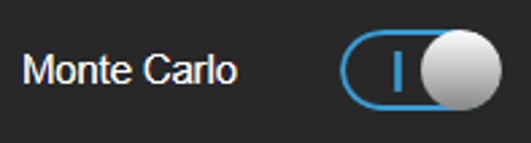# Monte Carlo Optimization

## Background

The VMAT optimization utilizes two ways of calculating doses in an intertwined manner:

• A beamlet based approximate dose calculation for high speed

• A forward dose calculation for high accuracy

Most of the necessary dose calculations during the optimization are performed with the approximate dose calculation. However, the forward dose calculation is used for correcting the dose values of the approximate dose calculation (by linear scaling). This enables, with limitations, simultaneously the best speed and accuracy. Therefore, the doses during optimization are always a hybrid of the approximate dose calculation and the forward dose calculation.

## Monte Carlo Toggle ButtonThe Monte Carlo toggle button serves two purposes:

1. To toggle the dose display from Pencil Beam to Monte Carlo mode (and vice versa). Thus, any visible dose (ACS views, DVH, risk analysis table, 3D dose displays) can be calculated with either of the two dose engines. Switching the toggle button only recalculates the dose distribution for the existing treatment plan with either Pencil Beam or Monte Carlo and does not (re-)optimize the current treatment plan.

2. To toggle the dose engine that is being used for the forward dose calculation during VMAT optimization. Therefore, when running the VMAT optimization, the following is true (the times in brackets give the typical time for one dose calculation):

• Monte Carlo toggle off: Beamlet based approximate dose (~ 1 msec), and Pencil Beam forward dose (~ 1 sec)

• Monte Carlo toggle on: Beamlet based approximate dose (~ 1 msec), and Monte Carlo forward dose (~ 1 min)

Art-No. 60919-40EN

Utgivningsdatum: 2019-05-22